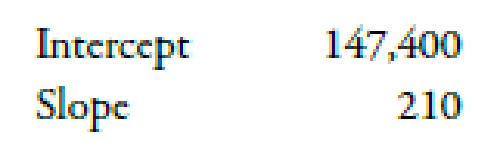Chapter 3, Problem 50E### Managerial Accounting: The Corners...

7th Edition
Maryanne M. Mowen + 2 others
ISBN: 9781337115773

#### Solutions

Chapter
Section### Managerial Accounting: The Corners...

7th Edition
Maryanne M. Mowen + 2 others
ISBN: 9781337115773
Textbook Problem
10 views

# The method of least squares was used to develop a cost equation to predict the cost of receiving purchased parts at a video game manufacturer. Ninety-six data points from monthly data were used for the regression. The following computer output was received:The cost driver used was number of parts inspected.Method of Least Squares, Budgeted Time Period Is Different from Time Period Used to Generate ResultsRefer to the company information in Exercise 3-49.Required: 1. What is the cost formula for a year? 2. Using the cost formula from Requirement 1, predict the cost of parts inspection for a year in which 70,000 parts are inspected.

1.

To determine

Prepare the cost formula.

Explanation

Cost:

Cost can be defined as the cash and cash equivalent which is incurred against the products or its related services which will benefit the organization in the future. There are two types of costs that are fixed and variable costs.

The cost formula is,

Total Annual Receiving Cost=Intercept Cost×12 months+(Slope Rate×Number Of Parts Inspected)

Substitute $147,400 for intercept cost and$210 for slope rate in the above formula.

Total Annual Receiving Cost=Intercept Cost×12 months+(

2.

To determine

Calculate the cost of receiving for the year if 70,000 parts are inspected.

### Still sussing out bartleby?

Check out a sample textbook solution.

See a sample solution

#### The Solution to Your Study Problems

Bartleby provides explanations to thousands of textbook problems written by our experts, many with advanced degrees!

Get Started